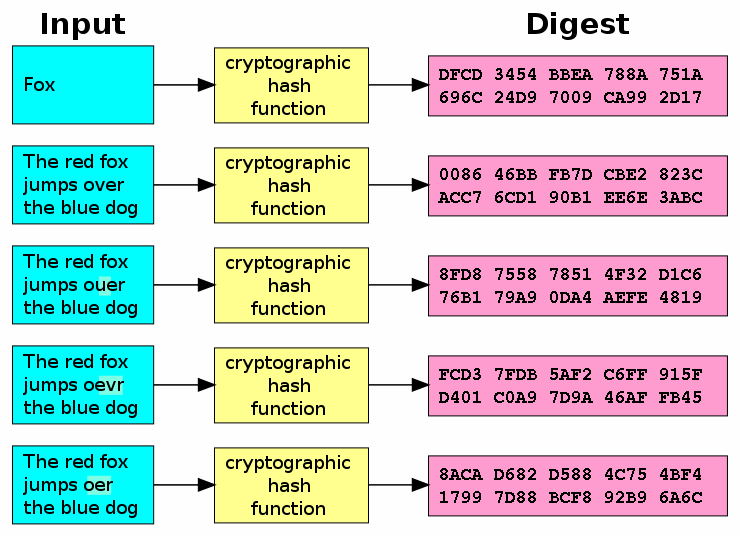Explore BrainMass
Share

# Hashing

Hashing is a way to map 'keys' (data of any type) to slots in a table of a set length. The key is put through a hash function to return a value, known as the hash valuehash code, hash sum, checksum or simply hash which is what is actually stored, instead of the data itself. The nature of a secure hashing function should be such that it is near impossible to work backwards from the hash value to find the original data value - this makes hashing ideal for storing passwords without storing the actual plaintext password. Another common use is shortening data since the output of a hash function must is of a fixed length. Cryptography also makes use of hashing as it's difficult to fake a hash value in order to hide malicious data. That is the whole idea behind the Pretty Good Privacy data validation algorithm. There are many other uses of hashing in real-world computer science too, so it is an important area to understand.

In a hash table, the has function outputs are stored associatively. This means the rows can be searched in constant time, and then that row's value used as a reference to point back to the key that holds the information you actually need. Since following the reference is also doable in constant time, this makes for a much faster search than iterating through a list, or many types of tree. A good hash function is referentially transparent. An obstacle to this fast searching is if two data values hash to the same slot in the hash table - called a collision. To deal with this, many programming languages have a way to override hash functions for an object.In any deterministic hasing function, there will be a set of keys that hashes to the same slot, causing collisions that degrade the usefulness of the table. To avoid this, one can use universal hashing which is when you select a hashing function from a family of such functions at random each time. Individually, they have the same limits as a deterministic function, but when picked at random from their family, you can evade most collisions and deny any attackers hoping to use that feature of a poorly-designed hashing table against you.

© BrainMass Inc. brainmass.com September 17, 2019, 6:25 am ad1c9bdddf

### Java Hashtables

We are working on hashtables and I am trying to figure out a real life example that could be solved with hashtables to jtree as well as some type of array or jlist. I just need some opinions or examples of this part please. Here is an exercise I do not understand in this chapter. Observe the following code, which create

### Email and Key Encryption

P18. Suppose Alice wants to send an e-mail to Bob. Bob has a public-private key pair, and Alice has Bob's certificate. But Alice does not have a public, private key pair. Alice and Bob (and the entire world) share the same hash function. a. In this situation, is it possible to design a scheme so that Bob can verify that Alice

### Given the URL hashing scheme, determine the caches to which requests for different URLs will be directed.

Suppose an institution has four caches, referred to as cache-0, cache-1, cache-2 and cache-3. Suppose that the URL hashing scheme operates as follows: (1) only the host name of the URL is used. (2) all non-alphabetic characters are ignored. (3) each alphabetic character is assigned a number corresponding to its position in th

### Encryption and Cryptography

This solution provides the learner with a short discussion on the concept of cryptography and the different aspects and functions that are provided by using encryption. Further, this solution provides some direct examples on how the different functions that are provided by the use of encryption can be applied to specific busines

### RSA signature

For RSA signature, let p = 17 and q = 43. Create a digital signature for the message m = 161, where the hashing function is the identity function and the computation at the signer's side is performed by the Chinese Remainder Theory. d = 5

### Excel 2003 Multiple Choice Questions

1. Cell E23 has a date value and you wish to place that date on an invoice prefaced with the text located in B15. What is the command to do that? (This question is from Chapter 6.) a. =B15&E23 b. =proper(B15)&" "&text(E23,"mmmm dd, yyyy") c. B15&" "&E23 d. =join(B15&E23) e. =proper(B15)&" "&proper(E23) 2. To

### quadratic searching - hashing

H(x) is a hash function performed on an identifier x. Show that if quadratic searching is carried out in the sequence (h(x) + q^2), (h(x) + (q-1)^2), ..., (h(x) + 1), h(x), (h(x) - 1), ..., (h(x) - q^2) with q = (b-1)/2, then the address difference % b between successive buckets being examined is b-2, b-4, b-6, ..., 5, 3, 1,